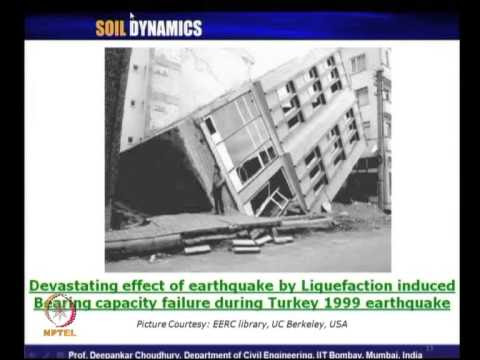### Soil DynamicsThe basic course in soil mechanics/geotechnical engineering generally introduces the fundamental concepts, principles and applications of soil as engineering material with properties under static loading. This course on “Soil Dynamics” discusses about the behaviour and properties/response of soil as a material which is subjected to various types of dynamic or cyclic time-dependent loadings.
Also the design and analysis for machine foundations come along with this course to consider the dynamic properties of both soil and foundation as combined mass. Behaviour of various geotechnical structures such as shallow and deep foundations, retaining structures, slopes, sub grade soil below railway, pavement, runway due to various types of time-dependent dynamic loading are discussed here along with the reference to design code provisions.
Phenomena like liquefaction and lateral spreading of soil are also discussed. The advanced course material on soil dynamics will be very useful to undergraduate students, post-graduate students, researchers, teachers and practitioners.  A number of selected problems are solved to illustrate the concepts clearly.
Contents:
Introduction to soil dynamics, Fundamentals of vibration theory, Wave propagation, Dynamic soil properties and liquefaction, Machine foundations, Dynamic soil improvement techniques, Dynamic soil-structure interaction.
 Sl.No. Module Contents No. ofHours 1. Introduction Scope and objective; Nature and types of dynamic loading; Importance of soil dynamics 01 2. Vibration theory Vibration of elementary systems; Degrees of freedom (SDOF and MDOF systems); Equation of motion for SDOF system; Types of vibrations; Earthquake excitation; Undamped and damped free vibrations; Torsional vibration; Critical damping; Decay of motion; Undamped and damped forced vibration; Constant force and rotating mass oscillators; Dynamic magnification factor; Transmissibility ratio; Non-harmonic, arbitrary, impact and other types of forced vibrations; Duhamel’s integral; Taxing of vehicles on uneven roads; Vibration isolation; Vibration measuring instruments; Equation of motion for MDOF system. 12 3. Wave Propagation Longitudinal and torsional waves in infinitely long rod; Solution for one-dimensional and three-dimensional equations of motion; Waves in semi-infinite body; Waves in layered medium; Earthquake waves – P-wave, S-wave, Rayleigh wave and Love wave; Locating earthquake's epicenter. 05 4. Dynamic Soil Properties Stresses in soil element; Determination of dynamic soil properties; Field tests; Laboratory tests; Model tests; Stress-strain behavior of cyclically loaded soils; Estimation of shear modulus; Modulus reduction curve; Damping ratio; Linear, equivalent-linear and non-linear models; Ranges and applications of dynamic soil tests; Cyclic plate load test; Liquefaction; Screening and estimation of liquefaction; Simplified procedure for liquefaction estimation; Factor of safety; Cyclic stress ratio; Cyclic resistance ratio; CRR correlations with SPT, CPT, SASW test values. 08 5. Machine Foundations Types of machines; Basic design criteria; Methods of analysis; Mass-Spring-Dashpot model; Elastic-Half-Space theory; Tschebotarioff’s reduced natural frequency method; Types of foundations; Modes of vibrations; Vertical, sliding, torsional (yawing) and rocking (and pitching) modes of oscillations; Design guidelines as per codes; Typical design problems. 11 6. Soil Improvement Techniques Basic concept of soil improvement due to dynamic loading; Various methods; Mitigation of liquefaction 02 7. Dynamic Soil-Structure Interaction Dynamic earth pressures; Force and displacement based analysis; Pseudo-static and Pseudo-dynamic analysis; Guidelines of various design codes; Dynamic analyses of various geotechnical structures like retaining wall, soil slope, railway subgrade and ballast using MSD model. 04